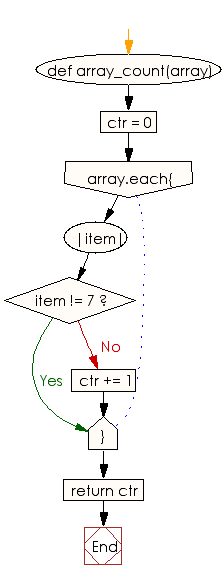﻿ Ruby Basic exercises: Check whether one of the first 5 elements in a given array of integers is a 7 - w3resource# Ruby Basic Exercises: Check whether one of the first 5 elements in a given array of integers is a 7

## Ruby Basic: Exercise-42 with Solution

Write a Ruby program to check whether one of the first 5 elements in a given array of integers is a 7. The array length may be less than 5.

Ruby Code:

``````def array_count(array)
ctr = 0
array.each{|item| ctr += 1 unless item != 7}
return ctr
end

print array_count([1, 2, 6, 4, 9]),"\n"
print array_count([1, 2, 5, 7, 9]),"\n"
print array_count([0, 2, 5, 7])
``````

Output:

```0
1
1
```

Flowchart:Ruby Code Editor: